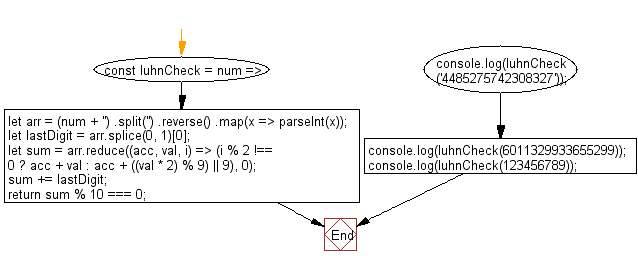# JavaScript: Implement the Luhn Algorithm used to validate a variety of identification numbers

## JavaScript fundamental (ES6 Syntax): Exercise-80 with Solution

Write a JavaScript program to implement the Luhn Algorithm used to validate a variety of identification numbers, such as credit card numbers, IMEI numbers, National Provider Identifier numbers etc.

• Use String.prototype.split(''), Array.prototype.reverse() and Array.prototype.map() in combination with parseInt() to obtain an array of digits.
• Use Array.prototype.splice(0, 1) to obtain the last digit.
• Use Array.prototype.reduce() to implement the Luhn Algorithm.
• Return true if sum is divisible by 10, false otherwise.

Sample Solution:

JavaScript Code:

``````//#Source https://bit.ly/2neWfJ2
const luhnCheck = num => {
let arr = (num + '')
.split('')
.reverse()
.map(x => parseInt(x));
let lastDigit = arr.splice(0, 1);
let sum = arr.reduce((acc, val, i) => (i % 2 !== 0 ? acc + val : acc + ((val * 2) % 9) || 9), 0);
sum += lastDigit;
return sum % 10 === 0;
};
console.log(luhnCheck('4485275742308327'));
console.log(luhnCheck(6011329933655299));
console.log(luhnCheck(123456789));
```
```

Sample Output:

```true
false
false
```

Flowchart:Live Demo:

See the Pen javascript-basic-exercise-80-1 by w3resource (@w3resource) on CodePen.

Improve this sample solution and post your code through Disqus

What is the difficulty level of this exercise?

Test your Programming skills with w3resource's quiz.

﻿

## JavaScript: Tips of the Day

Chunks an array into n smaller arrays

Example:

```const tips_chunkIntoN = (arr, n) => {
const size = Math.ceil(arr.length / n);
return Array.from({ length: n }, (v, i) =>
arr.slice(i * size, i * size + size)
);
}
console.log(tips_chunkIntoN([1, 2, 3, 4, 5, 6, 7,8], 4));
```

Output:

```[[1,2],[3,4],[5,6],[7,8]]
```